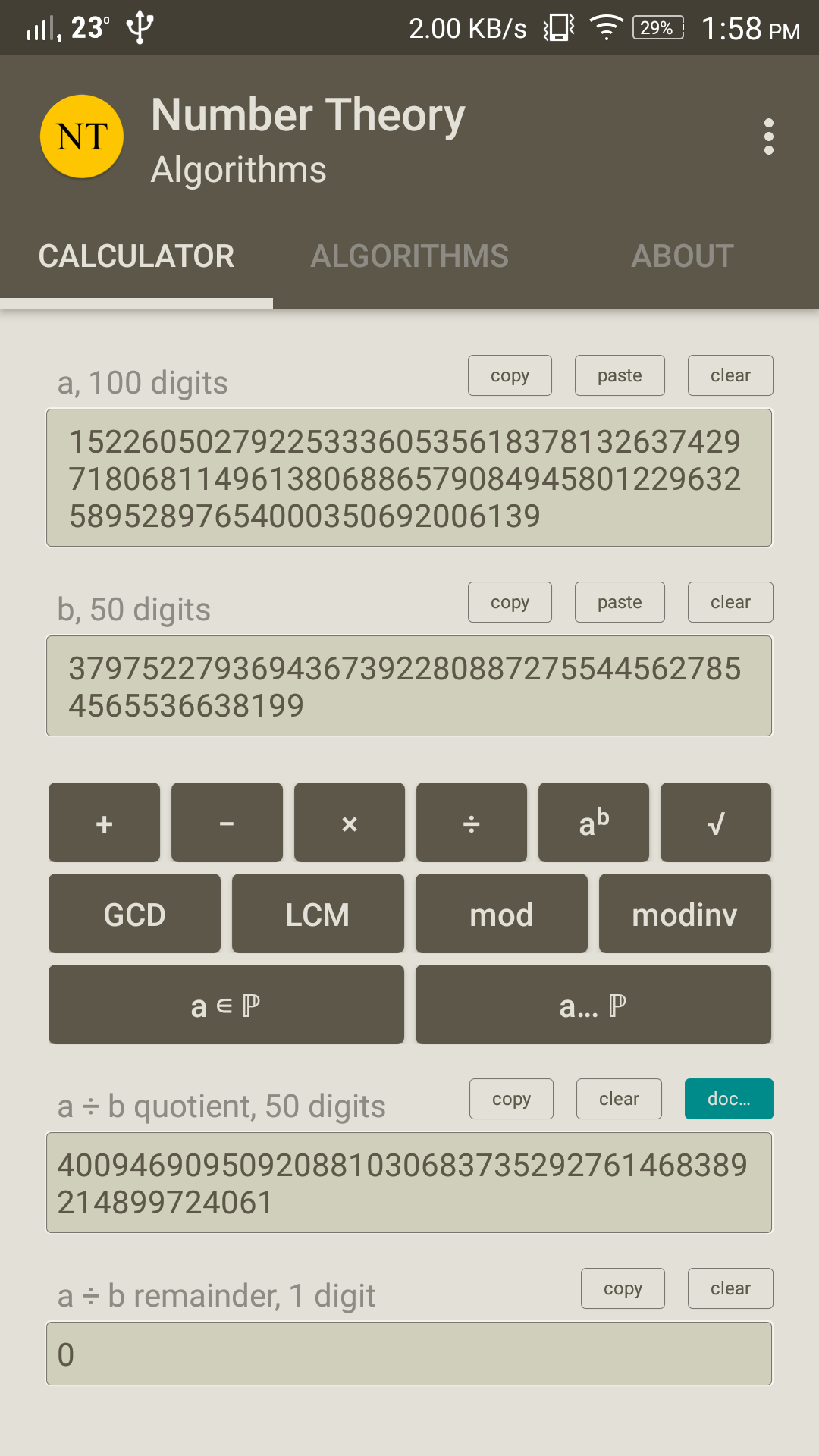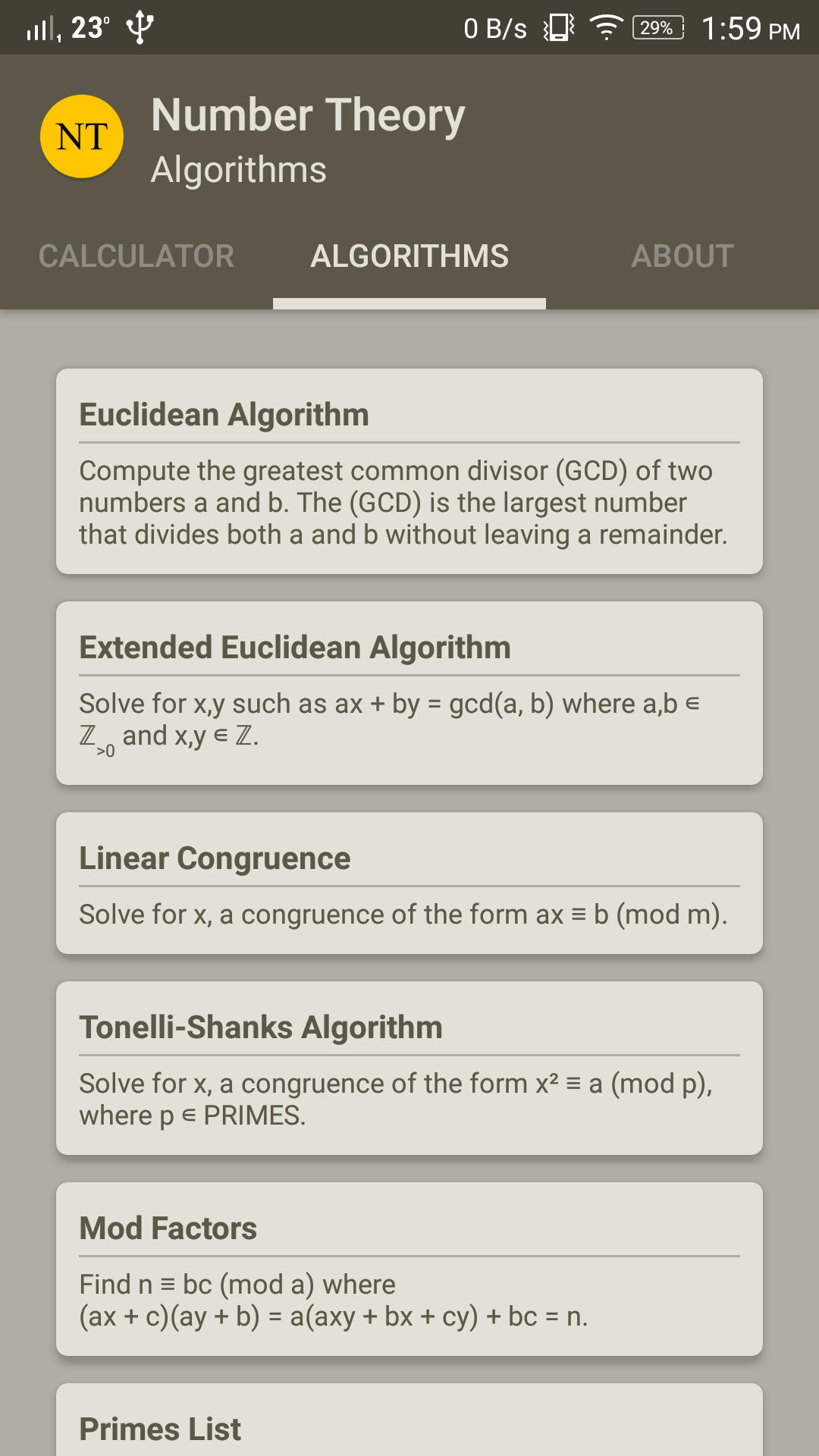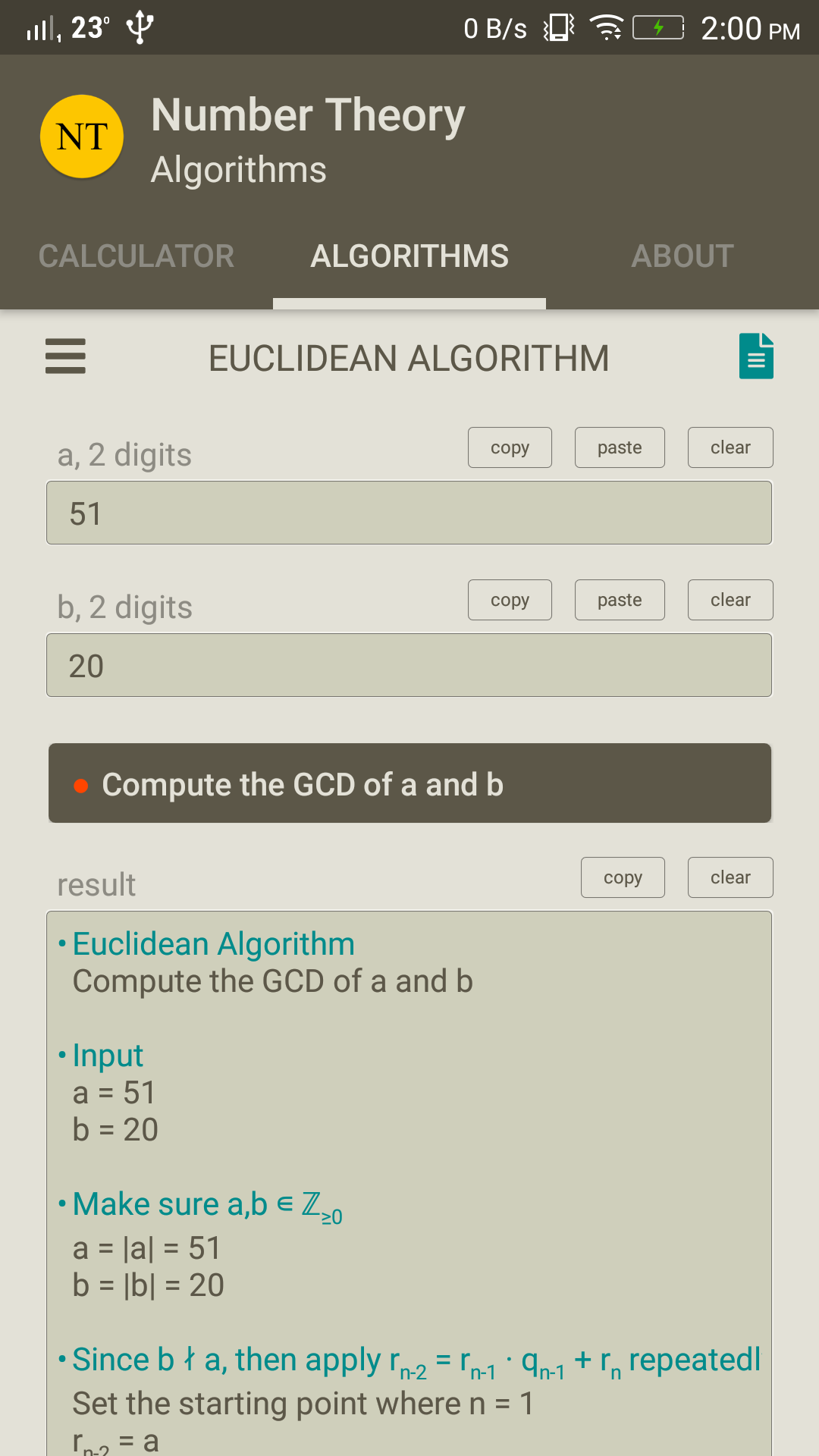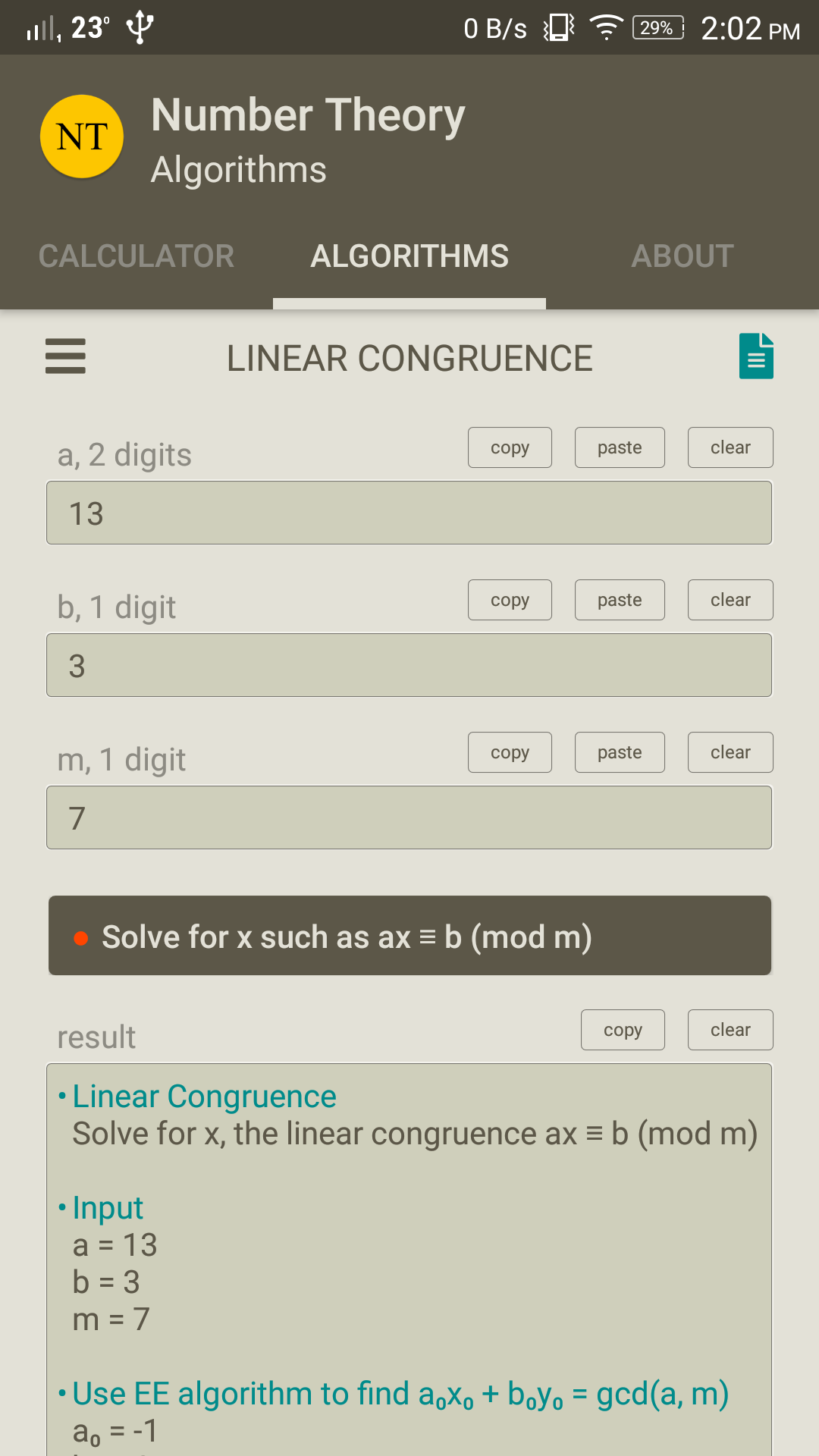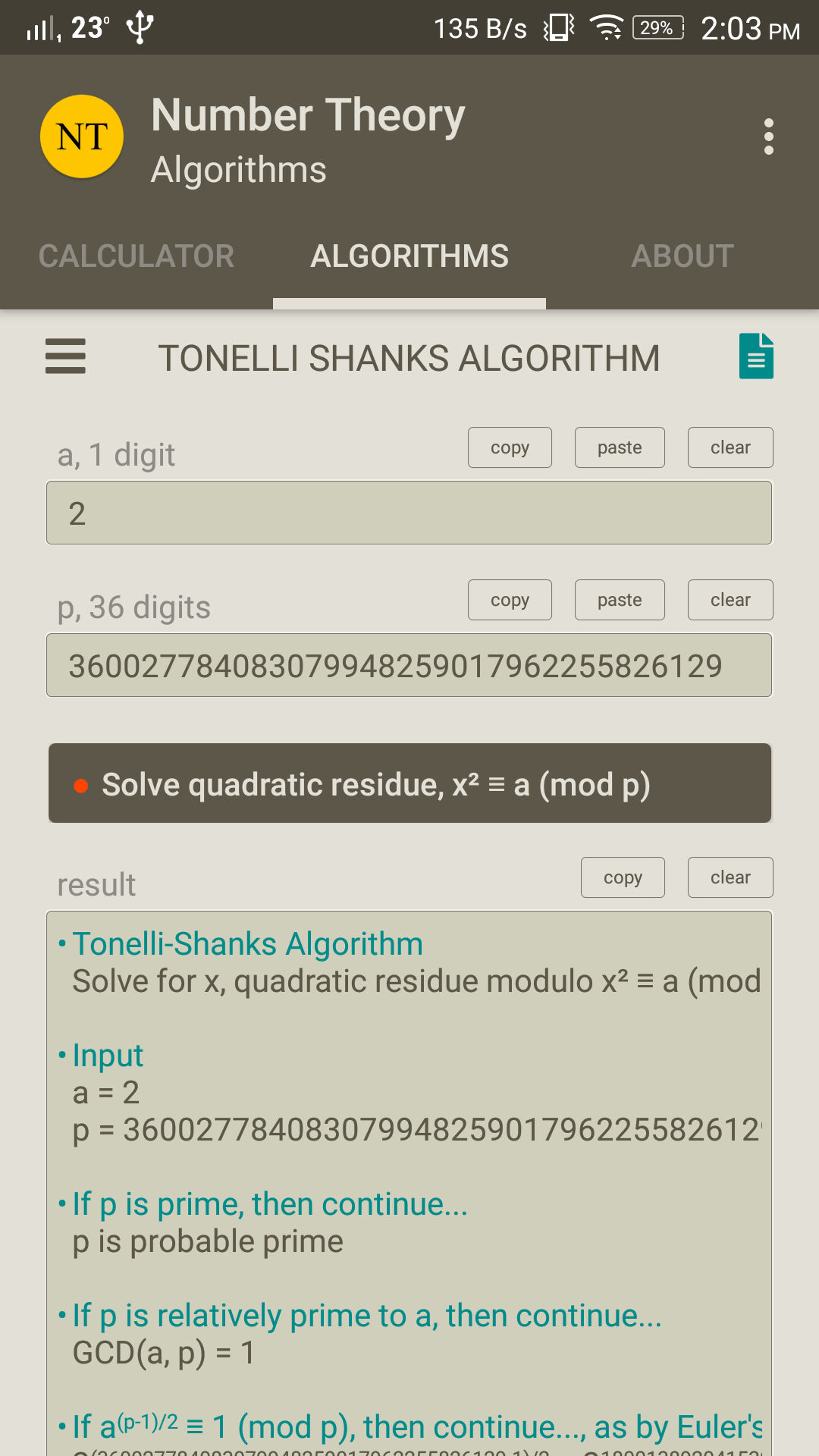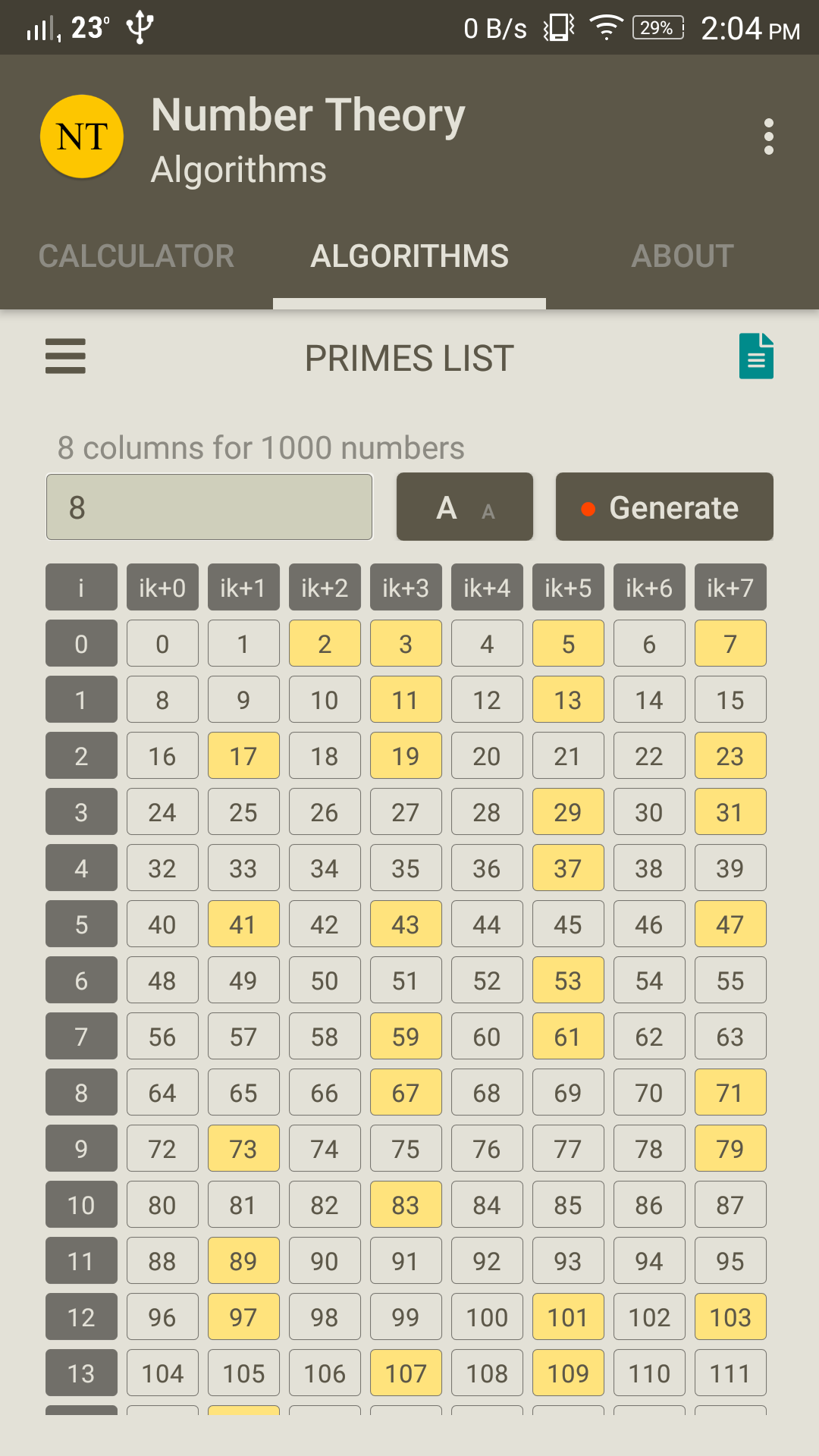﻿ Number Theory Algorithms

## Home / Number Theory Algorithms#### Number Theory Algorithms

Big integer calculator & some number theory algorithms in practise.

### Info

#### Calculator

• Add two big integer numbers.
• Subtract two big integer numbers.
• Multiply two big integer numbers.
• Divide two big integer numbers.
• Power of a big integer number (performance is based on the device).
• Root of a big integer number (performance is based on the device).
• GCD of two big integer numbers.
• LCM of two big integer numbers.
• Modulo of two big integer numbers.
• Modulo inverse of two big integer numbers.
• Check a big integer if is probable prime.
• Next probable prime to a big integer.

#### Algorithms

• Euclidean Algorithm, compute the greatest common divisor (GCD) of two numbers a and b.
• Extended Euclidean Algorithm, solve for x, y such as ax + by = gcd(a, b).
• Linear Congruence, Solve for x, a congruence of the form ax ≡ b (mod m).
• Tonelli-Shanks Algorithm, calculate quadratic residue modulo. Solve for x, a congruence of the form x² ≡ a (mod p) where p is prime.
• Mod-Factors, calculate modular possible factors for a given number and a modulo. Find n ≡ bc (mod a) where (ax + c)(ay + b) = a(axy + bx + cy) + bc = n.
• Primes List, list of primes ordered in columns.

### Screenshots